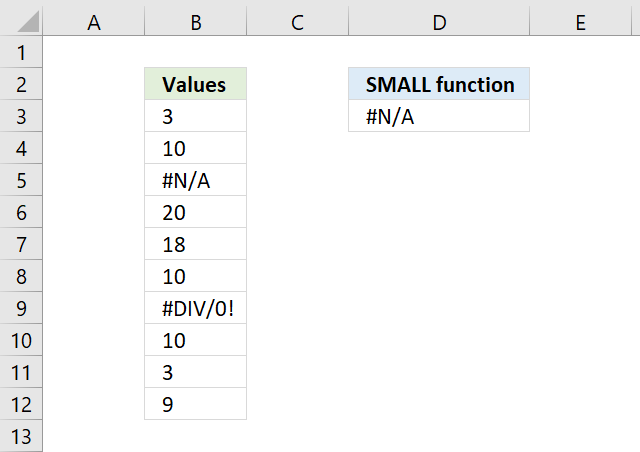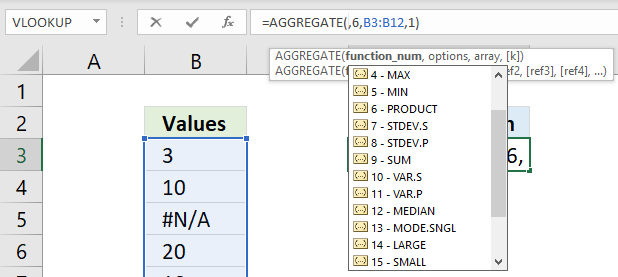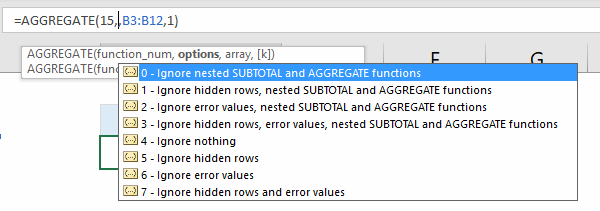Author: Oscar Cronquist Article last updated on July 09, 2022The image above shows you a formula in cell D3 that tries to get the smallest number from cell range B3:B12 but it returns an error. This happens if the cell range contains at least one error value.

Formula in cell D3:

=SMALL(B3:B12,1)

The SMALL function ignores text and boolean values but not error values, however, the AGGREGATE function lets you choose between a variety of functions (including SMALL function).

You also have the option to ignore error values, the second argument lets you specify this.

=AGGREGATE(15,6,B3:B12,1)

The AGGREGATE function contains these functions AVERAGE, COUNT, COUNTA, MAX, MIN, PRODUCT, STDEV.S, STDEV.P, SUM, VAR.S, VAR.P, MEDIAN, MODE.SNGL, LARGE, SMALL, PERCENTILE.INC, QUARTILE.INCPERCENTILE.EXC and QUARTILE.EXC.

You can see a list of available functions while entering the arguments in the function, see image below.The second argument has the following settings, I chose 6 - Ignore error values.The AGGREGATE function was introduced in Excel 2010, if you have an earlier Excel version then I recommend using the following array formula:

=SMALL(IFERROR(B3:B12,""),1)

To enter an array formula, type the formula in a cell then press and hold CTRL + SHIFT simultaneously, now press Enter once. Release all keys.

The formula bar now shows the formula with a beginning and ending curly bracket telling you that you entered the formula successfully. Don't enter the curly brackets yourself.

### Get the Excel fileHow-to-ignore-error-values-using-the-SMALL-function.xlsx